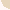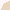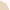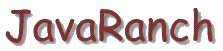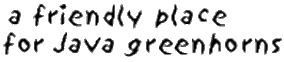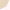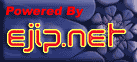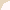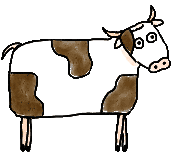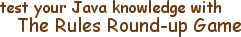# JavaRanch Rule Round-up Game

GOAL OF THE GAME: Herd all the cows into the pen by answering the questions correctly. Each time you answer correctly, a cow moves into the pen.

NUMBER OF ROUNDS: Depends on how many questions are in the pool. At the time of this writing, there are 161 questions in the pool.

NUMBER OF QUESTIONS PER ROUND: 12

SCORING:

After each incorrect answer you will see the correct answer.

At the end of each round you will see the number of questions and the number of correct answers as well as the percentage of correct answers.

When you have completed at least two rounds, you will also see the cumulative number of questions so far, the total number of correct answers, and the percentage of correct answers.

Good luck.

Page maintained by Marilyn de QueirozCopyright © 1998-2013 Paul Wheaton, Missoula, Montana